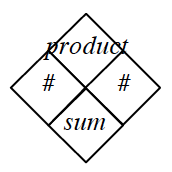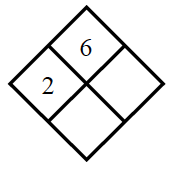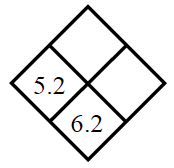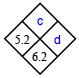### Home > MC1 > Chapter 3 > Lesson 3.1.1 > Problem3-8

3-8.

Copy and complete each of the Diamond Problems below.
The pattern used in the Diamond Problems is shown at right.

As shown in the diagram at right, the two numbers to the left and right of the diamond (marked with a $\#$ symbol) can be used to get the numbers on the top and bottom of the diamond. The number on the top of the diamond is the product of (answer you get when you multiply) the side numbers. The number on the bottom is the sum of (answer you get when you add) the side numbers.1.When you multiply the right side number by $2$ you get $6$! The bottom number is the sum of the two side numbers.

1.In the diamond above, the product (c) and number to the right (d) are missing. Use the numbers that are already filled in to find the missing pieces.Try finding the difference of the sum ($6.2$) and the number to the left ($5.2$). This value will be the number to the right (d).